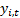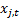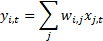/

### Blending Independent Components and Principal Components Analysis 2.2 Linear combination versus distribution mixtures

Next page

2.2          Linear combination versus distribution mixtures

Typically ICA assumes that the observed signals are a specific type of mixture of the underlying factors, namely a linear combination of these factors. The mixing coefficients applicable to this sort of mixture are the multipliers applied to each factor in the computation of the relevant output signal.

It is worth noting that the term ‘mixture’ has a variety of meanings in statistics. Here it is being used to mean that the output signals,, are derived from the input signals,using the following formula (whereindexes the observed values of a given signal, and so might correspond to points in time or space, andindexes the signals themselves):The term ‘mixture’ can also be used to describe a probability distribution for a random variable that is sequentially drawn with given probabilities from one of a number of different underlying distributions. The ‘mixing coefficients’ for such distribution mixtures relate to the probability of the given draw coming from the relevant underlying probability distribution. Such distribution mixtures can behave quite differently to the sorts of linear combination mixtures described above. We will explore the relevance of this latter type of mixture to portfolio risk management in Section 4.3.